# `garage.tf.algos`¶

Tensorflow implementation of reinforcement learning algorithms.

class DDPG(env_spec, policy, qf, replay_buffer, sampler, *, steps_per_epoch=20, n_train_steps=50, buffer_batch_size=64, min_buffer_size=int(10000.0), max_episode_length_eval=None, exploration_policy=None, target_update_tau=0.01, discount=0.99, policy_weight_decay=0, qf_weight_decay=0, policy_optimizer=tf.compat.v1.train.AdamOptimizer, qf_optimizer=tf.compat.v1.train.AdamOptimizer, policy_lr=_Default(0.0001), qf_lr=_Default(0.001), clip_pos_returns=False, clip_return=np.inf, max_action=None, reward_scale=1.0, name='DDPG')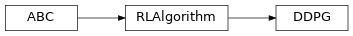A DDPG model based on https://arxiv.org/pdf/1509.02971.pdf.

DDPG, also known as Deep Deterministic Policy Gradient, uses actor-critic method to optimize the policy and reward prediction. It uses a supervised method to update the critic network and policy gradient to update the actor network. And there are exploration strategy, replay buffer and target networks involved to stabilize the training process.

Example

\$ python garage/examples/tf/ddpg_pendulum.py

Parameters
• env_spec (EnvSpec) – Environment specification.

• policy (Policy) – Policy.

• qf (object) – The q value network.

• replay_buffer (ReplayBuffer) – Replay buffer.

• sampler (garage.sampler.Sampler) – Sampler.

• steps_per_epoch (int) – Number of train_once calls per epoch.

• n_train_steps (int) – Training steps.

• buffer_batch_size (int) – Batch size of replay buffer.

• max_episode_length_eval (int or None) – Maximum length of episodes used for off-policy evaluation. If None, defaults to env_spec.max_episode_length.

• min_buffer_size (int) – The minimum buffer size for replay buffer.

• exploration_policy (ExplorationPolicy) – Exploration strategy.

• target_update_tau (float) – Interpolation parameter for doing the soft target update.

• policy_lr (float) – Learning rate for training policy network.

• qf_lr (float) – Learning rate for training q value network.

• discount (float) – Discount factor for the cumulative return.

• policy_weight_decay (float) – L2 regularization factor for parameters of the policy network. Value of 0 means no regularization.

• qf_weight_decay (float) – L2 regularization factor for parameters of the q value network. Value of 0 means no regularization.

• policy_optimizer (tf.compat.v1.train.Optimizer) – Optimizer for training policy network.

• qf_optimizer (tf.compat.v1.train.Optimizer) – Optimizer for training Q-function network.

• clip_pos_returns (bool) – Whether or not clip positive returns.

• max_action (float) – Maximum action magnitude.

• reward_scale (float) – Reward scale.

• name (str) – Name of the algorithm shown in computation graph.

train(trainer)

Obtain samplers and start actual training for each epoch.

Parameters

trainer (Trainer) – Experiment trainer, which provides services such as snapshotting and sampler control.

Returns

The average return in last epoch cycle.

Return type

float

class DQN(env_spec, policy, qf, replay_buffer, sampler, exploration_policy=None, steps_per_epoch=20, min_buffer_size=int(10000.0), buffer_batch_size=64, max_episode_length_eval=None, n_train_steps=50, qf_lr=0.001, qf_optimizer=tf.compat.v1.train.AdamOptimizer, discount=1.0, target_network_update_freq=5, grad_norm_clipping=None, double_q=False, reward_scale=1.0, name='DQN')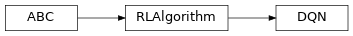DQN from https://arxiv.org/pdf/1312.5602.pdf.

Known as Deep Q-Network, it estimates the Q-value function by deep neural networks. It enables Q-Learning to be applied on high complexity environments. To deal with pixel environments, numbers of tricks are usually needed, e.g. skipping frames and stacking frames as single observation.

Parameters
• env_spec (EnvSpec) – Environment specification.

• policy (Policy) – Policy.

• qf (object) – The q value network.

• replay_buffer (ReplayBuffer) – Replay buffer.

• sampler (garage.sampler.Sampler) – Sampler.

• exploration_policy (ExplorationPolicy) – Exploration strategy.

• steps_per_epoch (int) – Number of train_once calls per epoch.

• min_buffer_size (int) – The minimum buffer size for replay buffer.

• buffer_batch_size (int) – Batch size for replay buffer.

• max_episode_length_eval (int or None) – Maximum length of episodes used for off-policy evaluation. If None, defaults to env_spec.max_episode_length.

• n_train_steps (int) – Training steps.

• qf_lr (float) – Learning rate for Q-Function.

• qf_optimizer (tf.compat.v1.train.Optimizer) – Optimizer for Q-Function.

• discount (float) – Discount factor for rewards.

• target_network_update_freq (int) – Frequency of updating target network.

• grad_norm_clipping (float) – Maximum clipping value for clipping tensor values to a maximum L2-norm. It must be larger than 0. If None, no gradient clipping is done. For detail, see docstring for tf.clip_by_norm.

• double_q (bool) – Bool for using double q-network.

• reward_scale (float) – Reward scale.

• name (str) – Name of the algorithm.

train(trainer)

Obtain samplers and start actual training for each epoch.

Parameters

trainer (Trainer) – Experiment trainer, which provides services such as snapshotting and sampler control.

Returns

The average return in last epoch cycle.

Return type

float

class ERWR(env_spec, policy, baseline, sampler, scope=None, discount=0.99, gae_lambda=1, center_adv=True, positive_adv=True, fixed_horizon=False, lr_clip_range=0.01, max_kl_step=0.01, optimizer=None, optimizer_args=None, policy_ent_coeff=0.0, use_softplus_entropy=False, use_neg_logli_entropy=False, stop_entropy_gradient=False, entropy_method='no_entropy', name='ERWR')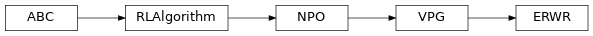Episodic Reward Weighted Regression .

Note

This does not implement the original RwR 2 that deals with “immediate reward problems” since it doesn’t find solutions that optimize for temporally delayed rewards.

1

Kober, Jens, and Jan R. Peters. “Policy search for motor primitives in robotics.” Advances in neural information processing systems. 2009.

2

Peters, Jan, and Stefan Schaal. “Using reward-weighted regression for reinforcement learning of task space control. ” Approximate Dynamic Programming and Reinforcement Learning, 2007. ADPRL 2007. IEEE International Symposium on. IEEE, 2007.

Parameters
• env_spec (EnvSpec) – Environment specification.

• policy (garage.tf.policies.StochasticPolicy) – Policy.

• baseline (garage.tf.baselines.Baseline) – The baseline.

• sampler (garage.sampler.Sampler) – Sampler.

• scope (str) – Scope for identifying the algorithm. Must be specified if running multiple algorithms simultaneously, each using different environments and policies.

• discount (float) – Discount.

• gae_lambda (float) – Lambda used for generalized advantage estimation.

• center_adv (bool) – Whether to rescale the advantages so that they have mean 0 and standard deviation 1.

• positive_adv (bool) – Whether to shift the advantages so that they are always positive. When used in conjunction with center_adv the advantages will be standardized before shifting.

• fixed_horizon (bool) – Whether to fix horizon.

• lr_clip_range (float) – The limit on the likelihood ratio between policies, as in PPO.

• max_kl_step (float) – The maximum KL divergence between old and new policies, as in TRPO.

• optimizer (object) – The optimizer of the algorithm. Should be the optimizers in garage.tf.optimizers.

• optimizer_args (dict) – The arguments of the optimizer.

• policy_ent_coeff (float) – The coefficient of the policy entropy. Setting it to zero would mean no entropy regularization.

• use_softplus_entropy (bool) – Whether to estimate the softmax distribution of the entropy to prevent the entropy from being negative.

• use_neg_logli_entropy (bool) – Whether to estimate the entropy as the negative log likelihood of the action.

• entropy_method (str) – A string from: ‘max’, ‘regularized’, ‘no_entropy’. The type of entropy method to use. ‘max’ adds the dense entropy to the reward for each time step. ‘regularized’ adds the mean entropy to the surrogate objective. See https://arxiv.org/abs/1805.00909 for more details.

• name (str) – The name of the algorithm.

train(trainer)

Obtain samplers and start actual training for each epoch.

Parameters

trainer (Trainer) – Experiment trainer, which rovides services such as snapshotting and sampler control.

Returns

The average return in last epoch cycle.

Return type

float

class NPO(env_spec, policy, baseline, sampler, scope=None, discount=0.99, gae_lambda=1, center_adv=True, positive_adv=False, fixed_horizon=False, pg_loss='surrogate', lr_clip_range=0.01, max_kl_step=0.01, optimizer=None, optimizer_args=None, policy_ent_coeff=0.0, use_softplus_entropy=False, use_neg_logli_entropy=False, stop_entropy_gradient=False, entropy_method='no_entropy', name='NPO')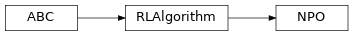Parameters
• env_spec (EnvSpec) – Environment specification.

• policy (garage.tf.policies.StochasticPolicy) – Policy.

• baseline (garage.tf.baselines.Baseline) – The baseline.

• sampler (garage.sampler.Sampler) – Sampler.

• scope (str) – Scope for identifying the algorithm. Must be specified if running multiple algorithms simultaneously, each using different environments and policies.

• discount (float) – Discount.

• gae_lambda (float) – Lambda used for generalized advantage estimation.

• center_adv (bool) – Whether to rescale the advantages so that they have mean 0 and standard deviation 1.

• positive_adv (bool) – Whether to shift the advantages so that they are always positive. When used in conjunction with center_adv the advantages will be standardized before shifting.

• fixed_horizon (bool) – Whether to fix horizon.

• pg_loss (str) – A string from: ‘vanilla’, ‘surrogate’, ‘surrogate_clip’. The type of loss functions to use.

• lr_clip_range (float) – The limit on the likelihood ratio between policies, as in PPO.

• max_kl_step (float) – The maximum KL divergence between old and new policies, as in TRPO.

• optimizer (object) – The optimizer of the algorithm. Should be the optimizers in garage.tf.optimizers.

• optimizer_args (dict) – The arguments of the optimizer.

• policy_ent_coeff (float) – The coefficient of the policy entropy. Setting it to zero would mean no entropy regularization.

• use_softplus_entropy (bool) – Whether to estimate the softmax distribution of the entropy to prevent the entropy from being negative.

• use_neg_logli_entropy (bool) – Whether to estimate the entropy as the negative log likelihood of the action.

• entropy_method (str) – A string from: ‘max’, ‘regularized’, ‘no_entropy’. The type of entropy method to use. ‘max’ adds the dense entropy to the reward for each time step. ‘regularized’ adds the mean entropy to the surrogate objective. See https://arxiv.org/abs/1805.00909 for more details.

• name (str) – The name of the algorithm.

Note

sane defaults for entropy configuration:
• entropy_method=’max’, center_adv=False, stop_gradient=True (center_adv normalizes the advantages tensor, which will significantly alleviate the effect of entropy. It is also recommended to turn off entropy gradient so that the agent will focus on high-entropy actions instead of increasing the variance of the distribution.)

train(trainer)

Obtain samplers and start actual training for each epoch.

Parameters

trainer (Trainer) – Experiment trainer, which rovides services such as snapshotting and sampler control.

Returns

The average return in last epoch cycle.

Return type

float

class PPO(env_spec, policy, baseline, sampler, scope=None, discount=0.99, gae_lambda=1, center_adv=True, positive_adv=False, fixed_horizon=False, lr_clip_range=0.01, max_kl_step=0.01, optimizer=None, optimizer_args=None, policy_ent_coeff=0.0, use_softplus_entropy=False, use_neg_logli_entropy=False, stop_entropy_gradient=False, entropy_method='no_entropy', name='PPO')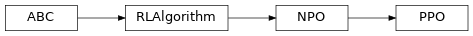Proximal Policy Optimization.

Parameters
• env_spec (EnvSpec) – Environment specification.

• policy (garage.tf.policies.StochasticPolicy) – Policy.

• baseline (garage.tf.baselines.Baseline) – The baseline.

• sampler (garage.sampler.Sampler) – Sampler.

• scope (str) – Scope for identifying the algorithm. Must be specified if running multiple algorithms simultaneously, each using different environments and policies.

• discount (float) – Discount.

• gae_lambda (float) – Lambda used for generalized advantage estimation.

• center_adv (bool) – Whether to rescale the advantages so that they have mean 0 and standard deviation 1.

• positive_adv (bool) – Whether to shift the advantages so that they are always positive. When used in conjunction with center_adv the advantages will be standardized before shifting.

• fixed_horizon (bool) – Whether to fix horizon.

• lr_clip_range (float) – The limit on the likelihood ratio between policies, as in PPO.

• max_kl_step (float) – The maximum KL divergence between old and new policies, as in TRPO.

• optimizer (object) – The optimizer of the algorithm. Should be the optimizers in garage.tf.optimizers.

• optimizer_args (dict) – The arguments of the optimizer.

• policy_ent_coeff (float) – The coefficient of the policy entropy. Setting it to zero would mean no entropy regularization.

• use_softplus_entropy (bool) – Whether to estimate the softmax distribution of the entropy to prevent the entropy from being negative.

• use_neg_logli_entropy (bool) – Whether to estimate the entropy as the negative log likelihood of the action.

• entropy_method (str) – A string from: ‘max’, ‘regularized’, ‘no_entropy’. The type of entropy method to use. ‘max’ adds the dense entropy to the reward for each time step. ‘regularized’ adds the mean entropy to the surrogate objective. See https://arxiv.org/abs/1805.00909 for more details.

• name (str) – The name of the algorithm.

train(trainer)

Obtain samplers and start actual training for each epoch.

Parameters

trainer (Trainer) – Experiment trainer, which rovides services such as snapshotting and sampler control.

Returns

The average return in last epoch cycle.

Return type

float

class REPS(env_spec, policy, baseline, sampler, discount=0.99, gae_lambda=1, center_adv=True, positive_adv=False, fixed_horizon=False, epsilon=0.5, l2_reg_dual=0.0, l2_reg_loss=0.0, optimizer=LBFGSOptimizer, optimizer_args=None, dual_optimizer=scipy.optimize.fmin_l_bfgs_b, dual_optimizer_args=None, name='REPS')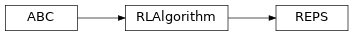Relative Entropy Policy Search.

References

 J. Peters, K. Mulling, and Y. Altun, “Relative Entropy Policy Search,”

Artif. Intell., pp. 1607-1612, 2008.

Example

\$ python garage/examples/tf/reps_gym_cartpole.py

Parameters
• env_spec (EnvSpec) – Environment specification.

• policy (garage.tf.policies.StochasticPolicy) – Policy.

• baseline (garage.tf.baselines.Baseline) – The baseline.

• sampler (garage.sampler.Sampler) – Sampler.

• scope (str) – Scope for identifying the algorithm. Must be specified if running multiple algorithms simultaneously, each using different environments and policies.

• discount (float) – Discount.

• gae_lambda (float) – Lambda used for generalized advantage estimation.

• center_adv (bool) – Whether to rescale the advantages so that they have mean 0 and standard deviation 1.

• positive_adv (bool) – Whether to shift the advantages so that they are always positive. When used in conjunction with center_adv the advantages will be standardized before shifting.

• fixed_horizon (bool) – Whether to fix horizon.

• epsilon (float) – Dual func parameter.

• l2_reg_dual (float) – Coefficient for dual func l2 regularization.

• l2_reg_loss (float) – Coefficient for policy loss l2 regularization.

• optimizer (object) – The optimizer of the algorithm. Should be the optimizers in garage.tf.optimizers.

• optimizer_args (dict) – Arguments of the optimizer.

• dual_optimizer (object) – Dual func optimizer.

• dual_optimizer_args (dict) – Arguments of the dual optimizer.

• name (str) – Name of the algorithm.

train(trainer)

Obtain samplers and start actual training for each epoch.

Parameters

trainer (Trainer) – Experiment trainer, which provides services such as snapshotting and sampler control.

Returns

The average return in last epoch cycle.

Return type

float

class RL2(env_spec, episodes_per_trial, meta_batch_size, task_sampler, meta_evaluator, n_epochs_per_eval, **inner_algo_args)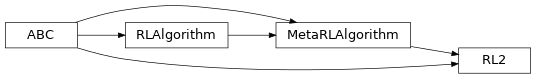RL^2.

Reference: https://arxiv.org/pdf/1611.02779.pdf.

When sampling for RL^2, there are more than one environments to be sampled from. In the original implementation, within each task/environment, all episodes sampled will be concatenated into one single episode, and fed to the inner algorithm. Thus, returns and advantages are calculated across the episode.

RL2Worker is required in sampling for RL2. See example/tf/rl2_ppo_halfcheetah.py for reference.

User should not instantiate RL2 directly. Currently garage supports PPO and TRPO as inner algorithm. Refer to garage/tf/algos/rl2ppo.py and garage/tf/algos/rl2trpo.py.

Parameters
• env_spec (EnvSpec) – Environment specification.

• episodes_per_trial (int) – Used to calculate the max episode length for the inner algorithm.

• meta_batch_size (int) – Meta batch size.

• meta_evaluator (MetaEvaluator) – Evaluator for meta-RL algorithms.

• n_epochs_per_eval (int) – If meta_evaluator is passed, meta-evaluation will be performed every n_epochs_per_eval epochs.

• inner_algo_args (dict) – Arguments for inner algorithm.

property policy

Policy to be used.

Type

Policy

property max_episode_length

Maximum length of an episode.

Type

int

train(trainer)

Obtain samplers and start actual training for each epoch.

Parameters

trainer (Trainer) – Experiment trainer, which provides services such as snapshotting and sampler control.

Returns

The average return in last epoch.

Return type

float

train_once(itr, episodes)

Perform one step of policy optimization given one batch of samples.

Parameters
• itr (int) – Iteration number.

• episodes (EpisodeBatch) – Batch of episodes.

Returns

Average return.

Return type

numpy.float64

get_exploration_policy()

Each time it is retrieved, this policy should only be evaluated in one task.

Returns

The policy used to obtain samples that are later used for

Return type

Policy

Parameters
• exploration_policy (Policy) – A policy which was returned from get_exploration_policy(), and which generated exploration_episodes by interacting with an environment. The caller may not use this object after passing it into this method.

• exploration_episodes (EpisodeBatch) – episodes to adapt to, generated by exploration_policy exploring the environment.

Returns

exploration_episodes.

Return type

Policy

class RL2PPO(meta_batch_size, task_sampler, env_spec, policy, baseline, sampler, episodes_per_trial, scope=None, discount=0.99, gae_lambda=1, center_adv=True, positive_adv=False, fixed_horizon=False, lr_clip_range=0.01, max_kl_step=0.01, optimizer_args=None, policy_ent_coeff=0.0, use_softplus_entropy=False, use_neg_logli_entropy=False, stop_entropy_gradient=False, entropy_method='no_entropy', meta_evaluator=None, n_epochs_per_eval=10, name='PPO')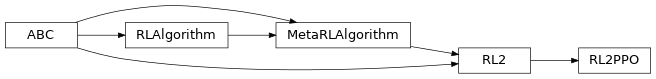Proximal Policy Optimization specific for RL^2.

See https://arxiv.org/abs/1707.06347 for algorithm reference.

Parameters
• meta_batch_size (int) – Meta batch size.

• env_spec (EnvSpec) – Environment specification.

• policy (garage.tf.policies.StochasticPolicy) – Policy.

• baseline (garage.tf.baselines.Baseline) – The baseline.

• sampler (garage.sampler.Sampler) – Sampler.

• episodes_per_trial (int) – Used to calculate the max episode length for the inner algorithm.

• scope (str) – Scope for identifying the algorithm. Must be specified if running multiple algorithms simultaneously, each using different environments and policies.

• discount (float) – Discount.

• gae_lambda (float) – Lambda used for generalized advantage estimation.

• center_adv (bool) – Whether to rescale the advantages so that they have mean 0 and standard deviation 1.

• positive_adv (bool) – Whether to shift the advantages so that they are always positive. When used in conjunction with center_adv the advantages will be standardized before shifting.

• fixed_horizon (bool) – Whether to fix horizon.

• lr_clip_range (float) – The limit on the likelihood ratio between policies, as in PPO.

• max_kl_step (float) – The maximum KL divergence between old and new policies, as in TRPO.

• optimizer_args (dict) – The arguments of the optimizer.

• policy_ent_coeff (float) – The coefficient of the policy entropy. Setting it to zero would mean no entropy regularization.

• use_softplus_entropy (bool) – Whether to estimate the softmax distribution of the entropy to prevent the entropy from being negative.

• use_neg_logli_entropy (bool) – Whether to estimate the entropy as the negative log likelihood of the action.

• entropy_method (str) – A string from: ‘max’, ‘regularized’, ‘no_entropy’. The type of entropy method to use. ‘max’ adds the dense entropy to the reward for each time step. ‘regularized’ adds the mean entropy to the surrogate objective. See https://arxiv.org/abs/1805.00909 for more details.

• meta_evaluator (garage.experiment.MetaEvaluator) – Evaluator for meta-RL algorithms.

• n_epochs_per_eval (int) – If meta_evaluator is passed, meta-evaluation will be performed every n_epochs_per_eval epochs.

• name (str) – The name of the algorithm.

property policy

Policy to be used.

Type

Policy

property max_episode_length

Maximum length of an episode.

Type

int

train(trainer)

Obtain samplers and start actual training for each epoch.

Parameters

trainer (Trainer) – Experiment trainer, which provides services such as snapshotting and sampler control.

Returns

The average return in last epoch.

Return type

float

train_once(itr, episodes)

Perform one step of policy optimization given one batch of samples.

Parameters
• itr (int) – Iteration number.

• episodes (EpisodeBatch) – Batch of episodes.

Returns

Average return.

Return type

numpy.float64

get_exploration_policy()

Each time it is retrieved, this policy should only be evaluated in one task.

Returns

The policy used to obtain samples that are later used for

Return type

Policy

Parameters
• exploration_policy (Policy) – A policy which was returned from get_exploration_policy(), and which generated exploration_episodes by interacting with an environment. The caller may not use this object after passing it into this method.

• exploration_episodes (EpisodeBatch) – episodes to adapt to, generated by exploration_policy exploring the environment.

Returns

exploration_episodes.

Return type

Policy

class RL2TRPO(meta_batch_size, task_sampler, env_spec, policy, baseline, sampler, episodes_per_trial, scope=None, discount=0.99, gae_lambda=0.98, center_adv=True, positive_adv=False, fixed_horizon=False, lr_clip_range=0.01, max_kl_step=0.01, optimizer=None, optimizer_args=None, policy_ent_coeff=0.0, use_softplus_entropy=False, use_neg_logli_entropy=False, stop_entropy_gradient=False, kl_constraint='hard', entropy_method='no_entropy', meta_evaluator=None, n_epochs_per_eval=10, name='TRPO')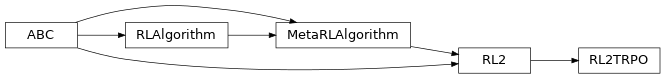Trust Region Policy Optimization specific for RL^2.

Parameters
• meta_batch_size (int) – Meta batch size.

• env_spec (EnvSpec) – Environment specification.

• policy (garage.tf.policies.StochasticPolicy) – Policy.

• baseline (garage.tf.baselines.Baseline) – The baseline.

• sampler (garage.sampler.Sampler) – Sampler.

• scope (str) – Scope for identifying the algorithm. Must be specified if running multiple algorithms simultaneously, each using different environments and policies.

• episodes_per_trial (int) – Used to calculate the max episode length for the inner algorithm.

• discount (float) – Discount.

• gae_lambda (float) – Lambda used for generalized advantage estimation.

• center_adv (bool) – Whether to rescale the advantages so that they have mean 0 and standard deviation 1.

• positive_adv (bool) – Whether to shift the advantages so that they are always positive. When used in conjunction with center_adv the advantages will be standardized before shifting.

• fixed_horizon (bool) – Whether to fix horizon.

• lr_clip_range (float) – The limit on the likelihood ratio between policies, as in PPO.

• max_kl_step (float) – The maximum KL divergence between old and new policies, as in TRPO.

• optimizer (object) – The optimizer of the algorithm. Should be the optimizers in garage.tf.optimizers.

• optimizer_args (dict) – The arguments of the optimizer.

• policy_ent_coeff (float) – The coefficient of the policy entropy. Setting it to zero would mean no entropy regularization.

• use_softplus_entropy (bool) – Whether to estimate the softmax distribution of the entropy to prevent the entropy from being negative.

• use_neg_logli_entropy (bool) – Whether to estimate the entropy as the negative log likelihood of the action.

• kl_constraint (str) – KL constraint, either ‘hard’ or ‘soft’.

• entropy_method (str) – A string from: ‘max’, ‘regularized’, ‘no_entropy’. The type of entropy method to use. ‘max’ adds the dense entropy to the reward for each time step. ‘regularized’ adds the mean entropy to the surrogate objective. See https://arxiv.org/abs/1805.00909 for more details.

• meta_evaluator (MetaEvaluator) – Evaluator for meta-RL algorithms.

• n_epochs_per_eval (int) – If meta_evaluator is passed, meta-evaluation will be performed every n_epochs_per_eval epochs.

• name (str) – The name of the algorithm.

property policy

Policy to be used.

Type

Policy

property max_episode_length

Maximum length of an episode.

Type

int

train(trainer)

Obtain samplers and start actual training for each epoch.

Parameters

trainer (Trainer) – Experiment trainer, which provides services such as snapshotting and sampler control.

Returns

The average return in last epoch.

Return type

float

train_once(itr, episodes)

Perform one step of policy optimization given one batch of samples.

Parameters
• itr (int) – Iteration number.

• episodes (EpisodeBatch) – Batch of episodes.

Returns

Average return.

Return type

numpy.float64

get_exploration_policy()

Each time it is retrieved, this policy should only be evaluated in one task.

Returns

The policy used to obtain samples that are later used for

Return type

Policy

Parameters
• exploration_policy (Policy) – A policy which was returned from get_exploration_policy(), and which generated exploration_episodes by interacting with an environment. The caller may not use this object after passing it into this method.

• exploration_episodes (EpisodeBatch) – episodes to adapt to, generated by exploration_policy exploring the environment.

Returns

exploration_episodes.

Return type

Policy

class TD3(env_spec, policy, qf, qf2, replay_buffer, sampler, *, target_update_tau=0.01, policy_weight_decay=0, qf_weight_decay=0, policy_optimizer=tf.compat.v1.train.AdamOptimizer, qf_optimizer=tf.compat.v1.train.AdamOptimizer, policy_lr=_Default(0.0001), qf_lr=_Default(0.001), clip_pos_returns=False, clip_return=np.inf, discount=0.99, max_episode_length_eval=None, max_action=None, name='TD3', steps_per_epoch=20, n_train_steps=50, buffer_batch_size=64, min_buffer_size=10000.0, reward_scale=1.0, exploration_policy_sigma=0.2, actor_update_period=2, exploration_policy_clip=0.5, exploration_policy=None)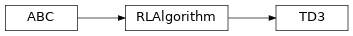Implementation of TD3.

Based on https://arxiv.org/pdf/1802.09477.pdf.

Example

\$ python garage/examples/tf/td3_pendulum.py

Parameters
• env_spec (EnvSpec) – Environment.

• policy (Policy) – Policy.

• qf (garage.tf.q_functions.QFunction) – Q-function.

• qf2 (garage.tf.q_functions.QFunction) – Q function to use

• replay_buffer (ReplayBuffer) – Replay buffer.

• sampler (garage.sampler.Sampler) – Sampler.

• target_update_tau (float) – Interpolation parameter for doing the soft target update.

• policy_lr (float) – Learning rate for training policy network.

• qf_lr (float) – Learning rate for training q value network.

• policy_weight_decay (float) – L2 weight decay factor for parameters of the policy network.

• qf_weight_decay (float) – L2 weight decay factor for parameters of the q value network.

• policy_optimizer (tf.compat.v1.train.Optimizer) – Optimizer for training policy network.

• qf_optimizer (tf.compat.v1.train.Optimizer) – Optimizer for training Q-function network.

• clip_pos_returns (boolean) – Whether or not clip positive returns.

• discount (float) – Discount factor for the cumulative return.

• max_episode_length_eval (int or None) – Maximum length of episodes used for off-policy evaluation. If None, defaults to env_spec.max_episode_length.

• max_action (float) – Maximum action magnitude.

• name (str) – Name of the algorithm shown in computation graph.

• steps_per_epoch (int) – Number of batches of samples in each epoch.

• n_train_steps (int) – Number of optimizations in each epoch cycle.

• buffer_batch_size (int) – Size of replay buffer.

• min_buffer_size (int) – Number of samples in replay buffer before first optimization.

• reward_scale (float) – Scale to reward.

• exploration_policy_sigma (float) – Action noise sigma.

• exploration_policy_clip (float) – Action noise clip.

• actor_update_period (int) – Action update period.

• exploration_policy (ExplorationPolicy) – Exploration strategy.

train(trainer)

Obtain samplers and start actual training for each epoch.

Parameters

trainer (Trainer) – Experiment trainer, which provides services such as snapshotting and sampler control.

Returns

The average return in last epoch cycle.

Return type

float

class TENPO(env_spec, policy, baseline, sampler, scope=None, discount=0.99, gae_lambda=1, center_adv=True, positive_adv=False, fixed_horizon=False, lr_clip_range=0.01, max_kl_step=0.01, optimizer=None, optimizer_args=None, policy_ent_coeff=0.0, encoder_ent_coeff=0.0, use_softplus_entropy=False, stop_ce_gradient=False, inference=None, inference_optimizer=None, inference_optimizer_args=None, inference_ce_coeff=0.0, name='NPOTaskEmbedding')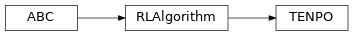Natural Policy Optimization with Task Embeddings.

See https://karolhausman.github.io/pdf/hausman17nips-ws2.pdf for algorithm reference.

Parameters
• env_spec (EnvSpec) – Environment specification.

• baseline (garage.tf.baselines.Baseline) – The baseline.

• sampler (garage.sampler.Sampler) – Sampler.

• scope (str) – Scope for identifying the algorithm. Must be specified if running multiple algorithms simultaneously, each using different environments and policies.

• discount (float) – Discount.

• gae_lambda (float) – Lambda used for generalized advantage estimation.

• center_adv (bool) – Whether to rescale the advantages so that they have mean 0 and standard deviation 1.

• positive_adv (bool) – Whether to shift the advantages so that they are always positive. When used in conjunction with center_adv the advantages will be standardized before shifting.

• fixed_horizon (bool) – Whether to fix horizon.

• lr_clip_range (float) – The limit on the likelihood ratio between policies, as in PPO.

• max_kl_step (float) – The maximum KL divergence between old and new policies, as in TRPO.

• optimizer (object) – The optimizer of the algorithm. Should be the optimizers in garage.tf.optimizers.

• optimizer_args (dict) – The arguments of the optimizer.

• policy_ent_coeff (float) – The coefficient of the policy entropy. Setting it to zero would mean no entropy regularization.

• encoder_ent_coeff (float) – The coefficient of the policy encoder entropy. Setting it to zero would mean no entropy regularization.

• use_softplus_entropy (bool) – Whether to estimate the softmax distribution of the entropy to prevent the entropy from being negative.

• inference (garage.tf.embeddings.StochasticEncoder) – A encoder that infers the task embedding from state trajectory.

• inference_optimizer (object) – The optimizer of the inference. Should be an optimizer in garage.tf.optimizers.

• inference_optimizer_args (dict) – The arguments of the inference optimizer.

• inference_ce_coeff (float) – The coefficient of the cross entropy of task embeddings inferred from task one-hot and state trajectory. This is effectively the coefficient of log-prob of inference.

• name (str) – The name of the algorithm.

train(trainer)

Obtain samplers and start actual training for each epoch.

Parameters

trainer (Trainer) – Trainer is passed to give algorithm the access to trainer.step_epochs(), which provides services such as snapshotting and sampler control.

Returns

The average return in last epoch cycle.

Return type

float

Get the embedding spec of the encoder.

Parameters

• latent_dim (int) – Latent dimension.

Returns

Encoder spec.

Return type

garage.InOutSpec

classmethod get_infer_spec(env_spec, latent_dim, inference_window_size)

Get the embedding spec of the inference.

Every inference_window_size timesteps in the trajectory will be used as the inference network input.

Parameters
• env_spec (garage.envs.EnvSpec) – Environment spec.

• latent_dim (int) – Latent dimension.

• inference_window_size (int) – Length of inference window.

Returns

Inference spec.

Return type

garage.InOutSpec

class TEPPO(env_spec, policy, baseline, sampler, scope=None, discount=0.99, gae_lambda=0.98, center_adv=True, positive_adv=False, fixed_horizon=False, lr_clip_range=0.01, max_kl_step=0.01, optimizer=None, optimizer_args=None, policy_ent_coeff=0.001, encoder_ent_coeff=0.001, use_softplus_entropy=False, stop_ce_gradient=False, inference=None, inference_optimizer=None, inference_optimizer_args=None, inference_ce_coeff=0.001, name='PPOTaskEmbedding')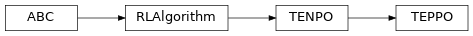Proximal Policy Optimization with Task Embedding.

See https://karolhausman.github.io/pdf/hausman17nips-ws2.pdf for algorithm reference.

Parameters
• env_spec (EnvSpec) – Environment specification.

• baseline (garage.tf.baselines.Baseline) – The baseline.

• sampler (garage.sampler.Sampler) – Sampler.

• scope (str) – Scope for identifying the algorithm. Must be specified if running multiple algorithms simultaneously, each using different environments and policies.

• discount (float) – Discount.

• gae_lambda (float) – Lambda used for generalized advantage estimation.

• center_adv (bool) – Whether to rescale the advantages so that they have mean 0 and standard deviation 1.

• positive_adv (bool) – Whether to shift the advantages so that they are always positive. When used in conjunction with center_adv the advantages will be standardized before shifting.

• fixed_horizon (bool) – Whether to fix horizon.

• lr_clip_range (float) – The limit on the likelihood ratio between policies, as in PPO.

• max_kl_step (float) – The maximum KL divergence between old and new policies, as in TRPO.

• optimizer (object) – The optimizer of the algorithm. Should be the optimizers in garage.tf.optimizers.

• optimizer_args (dict) – The arguments of the optimizer.

• policy_ent_coeff (float) – The coefficient of the policy entropy. Setting it to zero would mean no entropy regularization.

• encoder_ent_coeff (float) – The coefficient of the policy encoder entropy. Setting it to zero would mean no entropy regularization.

• use_softplus_entropy (bool) – Whether to estimate the softmax distribution of the entropy to prevent the entropy from being negative.

• inference (garage.tf.embedding.encoder.StochasticEncoder) – A encoder that infers the task embedding from a state trajectory.

• inference_optimizer (object) – The optimizer of the inference. Should be an optimizer in garage.tf.optimizers.

• inference_optimizer_args (dict) – The arguments of the inference optimizer.

• inference_ce_coeff (float) – The coefficient of the cross entropy of task embeddings inferred from task one-hot and state trajectory. This is effectively the coefficient of log-prob of inference.

• name (str) – The name of the algorithm.

train(trainer)

Obtain samplers and start actual training for each epoch.

Parameters

trainer (Trainer) – Trainer is passed to give algorithm the access to trainer.step_epochs(), which provides services such as snapshotting and sampler control.

Returns

The average return in last epoch cycle.

Return type

float

Get the embedding spec of the encoder.

Parameters

• latent_dim (int) – Latent dimension.

Returns

Encoder spec.

Return type

garage.InOutSpec

classmethod get_infer_spec(env_spec, latent_dim, inference_window_size)

Get the embedding spec of the inference.

Every inference_window_size timesteps in the trajectory will be used as the inference network input.

Parameters
• env_spec (garage.envs.EnvSpec) – Environment spec.

• latent_dim (int) – Latent dimension.

• inference_window_size (int) – Length of inference window.

Returns

Inference spec.

Return type

garage.InOutSpec

class TNPG(env_spec, policy, baseline, sampler, scope=None, discount=0.99, gae_lambda=0.98, center_adv=True, positive_adv=False, fixed_horizon=False, lr_clip_range=0.01, max_kl_step=0.01, optimizer=None, optimizer_args=None, policy_ent_coeff=0.0, use_softplus_entropy=False, use_neg_logli_entropy=False, stop_entropy_gradient=False, entropy_method='no_entropy', name='TNPG')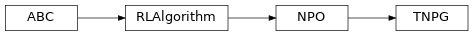Parameters
• env_spec (EnvSpec) – Environment specification.

• policy (garage.tf.policies.StochasticPolicy) – Policy.

• baseline (garage.tf.baselines.Baseline) – The baseline.

• sampler (garage.sampler.Sampler) – Sampler.

• scope (str) – Scope for identifying the algorithm. Must be specified if running multiple algorithms simultaneously, each using different environments and policies.

• discount (float) – Discount.

• gae_lambda (float) – Lambda used for generalized advantage estimation.

• center_adv (bool) – Whether to rescale the advantages so that they have mean 0 and standard deviation 1.

• positive_adv (bool) – Whether to shift the advantages so that they are always positive. When used in conjunction with center_adv the advantages will be standardized before shifting.

• fixed_horizon (bool) – Whether to fix horizon.

• lr_clip_range (float) – The limit on the likelihood ratio between policies, as in PPO.

• max_kl_step (float) – The maximum KL divergence between old and new policies, as in TRPO.

• optimizer (object) – The optimizer of the algorithm. Should be the optimizers in garage.tf.optimizers.

• optimizer_args (dict) – The arguments of the optimizer.

• policy_ent_coeff (float) – The coefficient of the policy entropy. Setting it to zero would mean no entropy regularization.

• use_softplus_entropy (bool) – Whether to estimate the softmax distribution of the entropy to prevent the entropy from being negative.

• use_neg_logli_entropy (bool) – Whether to estimate the entropy as the negative log likelihood of the action.

• entropy_method (str) – A string from: ‘max’, ‘regularized’, ‘no_entropy’. The type of entropy method to use. ‘max’ adds the dense entropy to the reward for each time step. ‘regularized’ adds the mean entropy to the surrogate objective. See https://arxiv.org/abs/1805.00909 for more details.

• name (str) – The name of the algorithm.

train(trainer)

Obtain samplers and start actual training for each epoch.

Parameters

trainer (Trainer) – Experiment trainer, which rovides services such as snapshotting and sampler control.

Returns

The average return in last epoch cycle.

Return type

float

class TRPO(env_spec, policy, baseline, sampler, scope=None, discount=0.99, gae_lambda=0.98, center_adv=True, positive_adv=False, fixed_horizon=False, lr_clip_range=0.01, max_kl_step=0.01, optimizer=None, optimizer_args=None, policy_ent_coeff=0.0, use_softplus_entropy=False, use_neg_logli_entropy=False, stop_entropy_gradient=False, kl_constraint='hard', entropy_method='no_entropy', name='TRPO')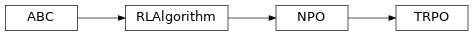Trust Region Policy Optimization.

Parameters
• env_spec (EnvSpec) – Environment specification.

• policy (garage.tf.policies.StochasticPolicy) – Policy.

• baseline (garage.tf.baselines.Baseline) – The baseline.

• sampler (garage.sampler.Sampler) – Sampler.

• scope (str) – Scope for identifying the algorithm. Must be specified if running multiple algorithms simultaneously, each using different environments and policies.

• discount (float) – Discount.

• gae_lambda (float) – Lambda used for generalized advantage estimation.

• center_adv (bool) – Whether to rescale the advantages so that they have mean 0 and standard deviation 1.

• positive_adv (bool) – Whether to shift the advantages so that they are always positive. When used in conjunction with center_adv the advantages will be standardized before shifting.

• fixed_horizon (bool) – Whether to fix horizon.

• lr_clip_range (float) – The limit on the likelihood ratio between policies, as in PPO.

• max_kl_step (float) – The maximum KL divergence between old and new policies, as in TRPO.

• optimizer (object) – The optimizer of the algorithm. Should be the optimizers in garage.tf.optimizers.

• optimizer_args (dict) – The arguments of the optimizer.

• policy_ent_coeff (float) – The coefficient of the policy entropy. Setting it to zero would mean no entropy regularization.

• use_softplus_entropy (bool) – Whether to estimate the softmax distribution of the entropy to prevent the entropy from being negative.

• use_neg_logli_entropy (bool) – Whether to estimate the entropy as the negative log likelihood of the action.

• kl_constraint (str) – KL constraint, either ‘hard’ or ‘soft’.

• entropy_method (str) – A string from: ‘max’, ‘regularized’, ‘no_entropy’. The type of entropy method to use. ‘max’ adds the dense entropy to the reward for each time step. ‘regularized’ adds the mean entropy to the surrogate objective. See https://arxiv.org/abs/1805.00909 for more details.

• name (str) – The name of the algorithm.

train(trainer)

Obtain samplers and start actual training for each epoch.

Parameters

trainer (Trainer) – Experiment trainer, which rovides services such as snapshotting and sampler control.

Returns

The average return in last epoch cycle.

Return type

float

class VPG(env_spec, policy, baseline, sampler, scope=None, discount=0.99, gae_lambda=1, center_adv=True, positive_adv=False, fixed_horizon=False, lr_clip_range=0.01, max_kl_step=0.01, optimizer=None, optimizer_args=None, policy_ent_coeff=0.0, use_softplus_entropy=False, use_neg_logli_entropy=False, stop_entropy_gradient=False, entropy_method='no_entropy', name='VPG')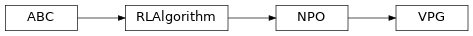Parameters
• env_spec (EnvSpec) – Environment specification.

• policy (garage.tf.policies.StochasticPolicy) – Policy.

• baseline (garage.tf.baselines.Baseline) – The baseline.

• sampler (garage.sampler.Sampler) – Sampler.

• scope (str) – Scope for identifying the algorithm. Must be specified if running multiple algorithms simultaneously, each using different environments and policies.

• discount (float) – Discount.

• gae_lambda (float) – Lambda used for generalized advantage estimation.

• center_adv (bool) – Whether to rescale the advantages so that they have mean 0 and standard deviation 1.

• positive_adv (bool) – Whether to shift the advantages so that they are always positive. When used in conjunction with center_adv the advantages will be standardized before shifting.

• fixed_horizon (bool) – Whether to fix horizon.

• lr_clip_range (float) – The limit on the likelihood ratio between policies, as in PPO.

• max_kl_step (float) – The maximum KL divergence between old and new policies, as in TRPO.

• optimizer (object) – The optimizer of the algorithm. Should be the optimizers in garage.tf.optimizers.

• optimizer_args (dict) – The arguments of the optimizer.

• policy_ent_coeff (float) – The coefficient of the policy entropy. Setting it to zero would mean no entropy regularization.

• use_softplus_entropy (bool) – Whether to estimate the softmax distribution of the entropy to prevent the entropy from being negative.

• use_neg_logli_entropy (bool) – Whether to estimate the entropy as the negative log likelihood of the action.

• entropy_method (str) – A string from: ‘max’, ‘regularized’, ‘no_entropy’. The type of entropy method to use. ‘max’ adds the dense entropy to the reward for each time step. ‘regularized’ adds the mean entropy to the surrogate objective. See https://arxiv.org/abs/1805.00909 for more details.

• name (str) – The name of the algorithm.

train(trainer)

Obtain samplers and start actual training for each epoch.

Parameters

trainer (Trainer) – Experiment trainer, which rovides services such as snapshotting and sampler control.

Returns

The average return in last epoch cycle.

Return type

float